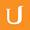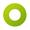## 13ps-04 Cover By Tree Solution

• 0:00 - 0:02
Alright, let's go ahead and take a look at this code.
• 0:02 - 0:06
We start out by grabbing the length of the n by n matrix
• 0:06 - 0:09
that we're interested in, and we store that in n.
• 0:09 - 0:14
Then we said v equaled -1; this is the vertex that we're currently looking at.
• 0:14 - 0:19
Now, for every element in the graph, every vertex in the graph,
• 0:19 - 0:22
we check if the assignment is none.
• 0:22 - 0:26
And if so, we set that to be the vertex that we are looking at right now.
• 0:26 - 0:31
Then we check, for each of those vertices, whether there is an edge
• 0:31 - 0:34
between that and any other vertices.
• 0:34 - 0:41
If there is, then either the assignment for i or j, the vertex we're starting at
• 0:41 - 0:44
and the vertex we're ending at, should be 1.
• 0:44 - 0:50
If both are zero, then this isn't going to work, so we return float infinity.
• 0:50 - 0:53
Now if we fall through checking every single element, and we don't get
• 0:53 - 0:58
float infinity as a return value, then if v is still equal to -1,
• 0:58 - 1:03
then we set the size equal to zero, and for every element in the assignment
• 1:03 -
that is equal to 1, we increase the size of the vertex cover until we return the total size.
Cím:
13ps-04 Cover By Tree Solution
Team:Udacity
Projekt:
CS313 - Theoretical Computer Science
Duration:
01:10Amara Bot hozzáadott egy fordítást

• Amara Bot